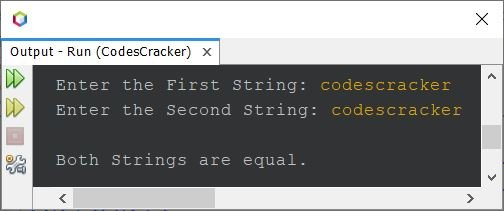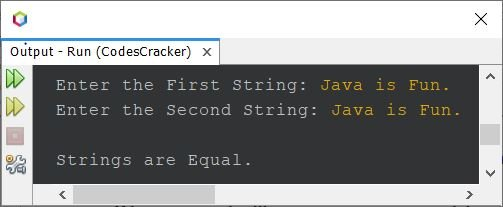# Java Program to Compare Two Strings

This article covers a program in Java that compares two strings entered by user at run-time of the program. Here are the list of programs covered in this article:

• Compare two strings using equals()
• Compare two strings using compareTo()
• Compare two strings character by character, without using equals() and compareTo()

## Compare Two Strings in Java using equals()

The question is, write a Java program that compares two given strings. The string must be received by user at run-time. The program given below is its answer:

```import java.util.Scanner;

public class CodesCracker
{
public static void main(String[] args)
{
String strOne, strTwo;
Scanner scan = new Scanner(System.in);

System.out.print("Enter the First String: ");
strOne = scan.nextLine();
System.out.print("Enter the Second String: ");
strTwo = scan.nextLine();

if(strOne.equals(strTwo))
System.out.println("\nBoth Strings are equal.");
else
System.out.println("\nBoth Strings are not equal.");
}
}```

The snapshot given below shows the sample run of above program with user input codescracker as both string:## Compare Two Strings in Java using compareTo()

To compare two strings using compareTo() method, just replace the condition of if statement. That is, replace the following code, from above program:

`if(strOne.equals(strTwo))`

with the code given below:

`if(strOne.compareTo(strTwo)==0)`

```import java.util.Scanner;

public class CodesCracker
{
public static void main(String[] args)
{
Scanner scan = new Scanner(System.in);

System.out.print("Enter the First String: ");
String strOne = scan.nextLine();
System.out.print("Enter the Second String: ");
String strTwo = scan.nextLine();

if(strOne.compareTo(strTwo) > 0)
System.out.println("\nThe String \"" +strOne+ "\" is larger than \"" +strTwo);
else if(strOne.compareTo(strTwo) < 0)
System.out.println("\nThe String \"" +strOne+ "\" is smaller than \"" +strTwo);
else
System.out.println("\nStrings are equal to each other.");
}
}```

Here is its sample run with user input codes as first and cracker as second string.## Compare Two Strings in Java - Character by Character

This program compares two strings in character by character manner. Also, this program does not uses equals() and compareTo() methods to do the job.

```import java.util.Scanner;

public class CodesCracker
{
public static void main(String[] args)
{
int k=0;
Scanner scan = new Scanner(System.in);

System.out.print("Enter the First String: ");
String strOne = scan.nextLine();
System.out.print("Enter the Second String: ");
String strTwo = scan.nextLine();

int strOneLen = strOne.length();
int strTwoLen = strTwo.length();

if(strOneLen == strTwoLen)
{
for(int i=0; i<strOneLen; i++)
{
if(strOne.charAt(i)==strTwo.charAt(i))
k++;
else
{
System.out.println("\nStrings are Unequal.");
break;
}
}
}
else
System.out.println("\nStrings are of Unequal size/length.");
if(k==strOneLen)
System.out.println("\nStrings are Equal.");
}
}```

Here is its sample run with user input Java is Fun. as both strings:#### Same Program in Other Languages

Java Online Test

« Previous Program Next Program »